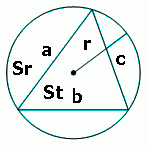homechevron_rightStudychevron_rightMathchevron_rightGeometry

# Circumcircle of a triangle

This online calculator determines the radius and area of the circumcircle of a triangle given the three sidesYet another triangle calculator, for those who needed radius of triangle circumcircle.
Radius can be found like this:

where S, area of triangle, can be found using Hero's formula

Calculator determines radius, and having radius, area of circumcircle, area of triange and area ratio - just for reference#### Circumcircle of a triangle

Digits after the decimal point: 2PLANETCALC, Circumcircle of a triangle